# Fermat's primality test facts for kids

Kids Encyclopedia Facts

Fermat's primality test is an algorithm. It can test if a given number p is probably prime. There is a flaw however: There are numbers that pass the test, and that are not prime. These numbers are called Carmichael numbers.

## Concept

Fermat's little theorem states that if p is prime and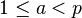$1 \le a < p$, then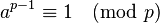$a^{p-1} \equiv 1 \pmod{p}$.

If we want to test if n is prime, then we can pick random a's in the interval and see if the equation above holds. If the equality does not hold for a value of a, then n is composite (not prime). If the equality does hold for many values of a, then we can say that n is probably prime, or a pseudoprime.

It may be in our tests that we do not pick any value for a such that the equality fails. Any a such that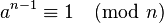$a^{n-1} \equiv 1 \pmod{n}$

when n is composite is known as a Fermat liar. If we do pick an a such that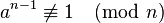$a^{n-1} \not\equiv 1 \pmod{n}$

then a is known as a Fermat witness for the compositeness of n.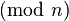$\pmod n$ is the modulo operation. Its result is what remains, if p is divided by n. As an example,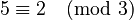$5 \equiv 2 \pmod 3$.

## What this test is used for

The RSA algorithm for public-key encryption can be done in such a way that it uses this test. This is useful in cryptography.Fermat's primality test Facts for Kids. Kiddle Encyclopedia.